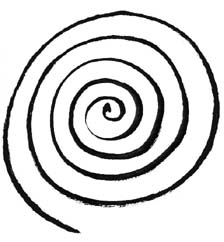# Spiral Review Quiz For Students! Test

9 Questions | Total Attempts: 300SettingsWhen it comes to math, you need to ensure that you take adequate practice to ensure that you do not lose all the things you have been able to understand so far. The quiz below is one of the spiral review quizzes that are designed to help a student with their math problems. Do give it a try and get some practice.

• 1.
#1b.
• A.

1.5

• B.

2.5

• C.

3

• D.

Not here

• 2.
#1c.
• A.

0

• B.

2

• C.

4

• D.

Not here

• 3.
#4
• A.

6

• B.

4

• C.

2

• D.

Not here

• 4.
#5.
• A.

3

• B.

-3

• C.

5

• D.

Not here

• 5.
#8
• A.

D: {1, 2, 3, 4, 5} R: {0, 2, 4, 6, 8} not a function

• B.

D: {-2, -1, 0, 1, 2} R: {4, 1, 0, 1, 4} yes, a function

• C.

D: {-3, -1, 0, 1, 3} R: {-4, 0, 2, 4} yes, a function

• D.

D: -3 < x < 3 R: -4 < y < 4 not a function

• 6.
#9
• A.

P > -4

• B.

P < 4

• C.

P < -4

• D.

P > 4

• 7.
#10.
• A.

K>-2

• B.

K

• C.

K>2

• D.

Not here

• 8.
#13
• A.

H(-6) = 3; h(9) = 7

• B.

H(-6) = -3; h(9) = 4

• C.

H(-6) = -1; h(9) = -5

• D.

Not here

• 9.
#15.
• A.

X>0

• B.

All real numbers

• C.

No solution

• D.

Not here

Related TopicsBack to top